# Linux login without passwd, port forwarding, X11 forwarding

simply exec

$ssh-keygen press enter till the end, then exec$    ssh-copy-id  user@host      (from client to host, the host is the server you want to log in)

#### Port forwarding and X11 forwarding

When login, simple add  -L (port forwarding), and optional -X (X11 forwarding)

\$    ssh  -X -L    port: localhost: port   user@host

first port is the client machine’s localhost’s port

localhost is the server machine’s url , can be diff of localhost, e.g. google.com , where server machine’s internet access google.com, that is the idea of proxy, e.g. access google from China (client machine) login to server machine, forwarding server machine’s access of google to client machine’s localhost

second port is the port of the middle url (localhost in this case), if this port set to 80, it is the default webpage, where we do not need to specify port

# more visualization

Time series

image wrangling

In :
import pandas as pd
import matplotlib.pyplot as plt
% matplotlib inline
from yahoo_finance import Share
import numpy as np

In :
btx=Share('BTX')
df=pd.DataFrame(btx.get_historical('2016-01-01','2016-04-01'))
df['Date']=pd.to_datetime(df['Date'])
df.dtypes

Out:
Adj_Close            object
Close                object
Date         datetime64[ns]
High                 object
Low                  object
Open                 object
Symbol               object
Volume               object
dtype: object
In :
df.index=df['Date']
df=df.drop(['Date','Symbol'],axis=1)

In :
df.head(3)

Out:
Adj_Close Close High Low Open Volume
Date
2016-04-01 2.92 2.92 3.02 2.84 2.90 281100
2016-03-31 2.87 2.87 2.90 2.76 2.83 277800
2016-03-30 2.77 2.77 2.83 2.65 2.70 239900
In :
plt.plot(df.iloc[:,:-1])

Out:
<matplotlib.legend.Legend at 0x7f16d7c191d0>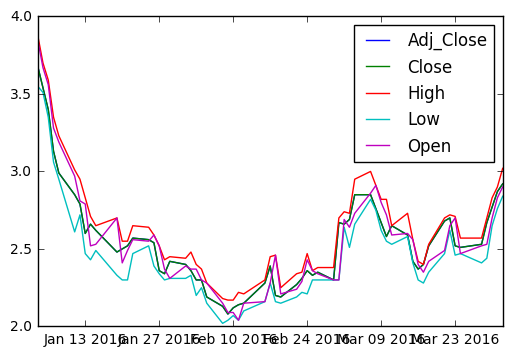## Plotting an inset view¶

In :
plt.plot(df['2016-01']['Open'])
plt.xticks(size=7, rotation=40)

plt.axes([0.55,0.4,0.3,0.45])
plt.plot(df['2016-02']['Open'],color='red')
plt.xticks(size=5, rotation=25)

Out:
(array([ 735997.,  736000.,  736003.,  736006.,  736009.,  736012.,
736015.,  736018.,  736021.]), <a list of 9 Text xticklabel objects>)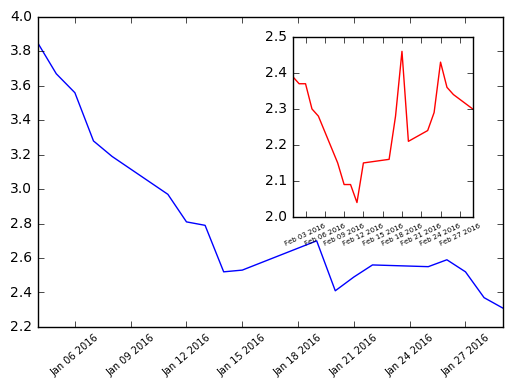## Cumulative Distribution Function from an image histogram¶

• A histogram of a continuous random variable is sometimes called a Probability Distribution Function (or PDF).
• The area under a PDF (a definite integral) is called a Cumulative Distribution Function (or CDF). The CDF quantifies the probability of observing certain pixel intensities.
• The histogram option cumulative=True permits viewing the CDF instead of the PDF.
In :
orig = plt.imread('cat.jpg')
print orig.shape
pixels = orig.flatten()
print len(pixels), pixels.max(), pixels.min()

(194, 259, 3)
150738 255 0


### plt.twinx()¶

• The command plt.twinx() allows two plots to be overlayed sharing the x-axis but with different scales on the y-axis.
In :
# Display a histogram of the pixels
plt.hist(pixels, bins=64, range=(0,256), normed=False,
color='red', alpha=0.3)

# Use plt.twinx() to overlay the CDF
plt.twinx()

# Display a cumulative histogram of the pixels

plt.hist(pixels, bins=64, range=(0,256), normed=True,cumulative=True,
color='blue', alpha=0.3)
plt.title('PDF & CDF (original image)')

plt.show()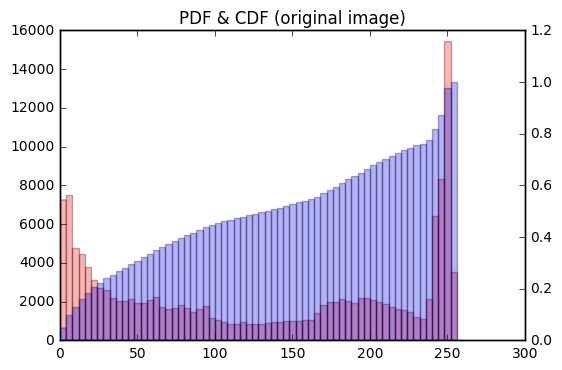## Equlize the image¶

• Histogram equalization is an image processing procedure that reassigns image pixel intensities. The basic idea is to use interpolation to map the original CDF of pixel intensities to a CDF that is almost a straight line. In essence, the pixel intensities are spread out and this has the practical effect of making a sharper, contrast-enhanced image. This is particularly useful in astronomy and medical imaging to help us see more features.

https://en.wikipedia.org/wiki/Histogram_equalization

In :
# Load the image into an array: image

# Flatten the image into 1 dimension: pixels
pixels = image.flatten()

# Generate a cumulative histogram
cdf, bins, patches = plt.hist(pixels, bins=256, range=(0,256), normed=True, cumulative=True)
new_pixels = np.interp(pixels, bins[:-1], cdf*255)

# Reshape new_pixels as a 2-D array: new_image
new_image = new_pixels.reshape(image.shape)

# Display the new image with 'gray' color map
plt.subplot(2,1,1)
plt.title('Equalized image')
plt.axis('off')
plt.imshow(new_image, cmap='gray')

# Generate a histogram of the new pixels
plt.subplot(2,1,2)
pdf = plt.hist(new_pixels, bins=64, range=(0,256), normed=False,
color='red', alpha=0.4)
plt.grid('off')

# Use plt.twinx() to overlay the CDF in the bottom subplot
plt.twinx()
plt.xlim((0,256))
plt.grid('off')

plt.title('PDF & CDF (equalized image)')

# Generate a cumulative histogram of the new pixels
cdf = plt.hist(new_pixels, bins=64, range=(0,256),
cumulative=True, normed=True,
color='blue', alpha=0.4)
plt.show()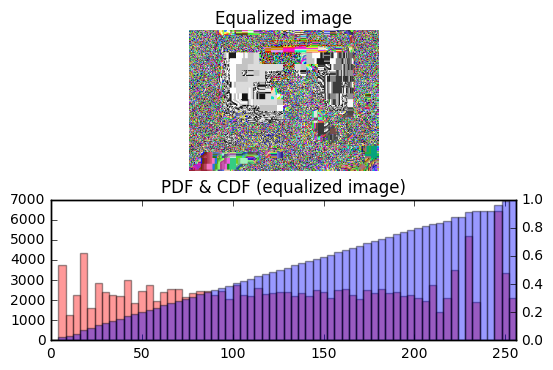## Extracting histograms from a color image¶

• The separate RGB (red-green-blue) channels will be extracted for you as two-dimensional arrays red, green, and blue respectively. You will plot three overlaid color histograms on common axes (one for each channel) in a subplot as well as the original image in a separate subplot.
In :
# Load the image into an array: image

# Display image in top subplot
plt.subplot(2,1,1)
plt.title('Original image')
plt.axis('off')
plt.imshow(image)

# Extract 2-D arrays of the RGB channels: red, blue, green
red, blue, green = image[:,:,0], image[:,:,1], image[:,:,2]

# Flatten the 2-D arrays of the RGB channels into 1-D
red_pixels = red.flatten()
blue_pixels = blue.flatten()
green_pixels = green.flatten()

# Overlay histograms of the pixels of each color in the bottom subplot
plt.subplot(2,1,2)
plt.title('Histograms from color image')
plt.xlim((0,256))
plt.hist(red_pixels, bins=64, normed=True, color='red', alpha=0.2)
plt.hist(blue_pixels, bins=64, normed=True, color='blue', alpha=0.2)
plt.hist(green_pixels, bins=64, normed=True, color='green', alpha=0.2)

# Display the plot
plt.show()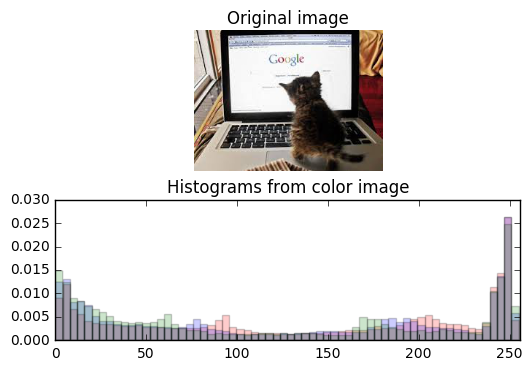## Extracting bivariate histograms from a color image¶

• Rather than overlaying univariate histograms of intensities in distinct channels, it is also possible to view the joint variation of pixel intensity in two different channels.
• The separate RGB (red-green-blue) channels will be extracted for you as one-dimensional arrays red_pixels, green_pixels, & blue_pixels respectively.
In :
# Load the image into an array: image

# Extract RGB channels and flatten into 1-D array
red, blue, green = image[:,:,0], image[:,:,1], image[:,:,2]
red_pixels = red.flatten()
blue_pixels = blue.flatten()
green_pixels = green.flatten()

# Generate a 2-D histogram of the red and green pixels
plt.subplot(2,2,1)
plt.grid('off')
plt.xticks(rotation=60)
plt.xlabel('red')
plt.ylabel('green')
plt.hist2d(red_pixels, green_pixels, bins=(32,32))

# Generate a 2-D histogram of the green and blue pixels
plt.subplot(2,2,2)
plt.grid('off')
plt.xticks(rotation=60)

plt.yticks(size=6)

plt.xlabel('green')
plt.ylabel('blue')
plt.hist2d(green_pixels, blue_pixels, bins=(32, 32))

# Generate a 2-D histogram of the blue and red pixels
plt.subplot(2,2,3)
plt.grid('off')
plt.xticks(rotation=60)

plt.xlabel('blue',size=5,color='r')
plt.ylabel('red')
plt.hist2d(blue_pixels, red_pixels, bins=(32, 32))

plt.subplot(2,2,4)
plt.grid('off')
plt.xticks(rotation=60)
plt.title('orig')
plt.imshow(image)

# Display the plot
plt.show()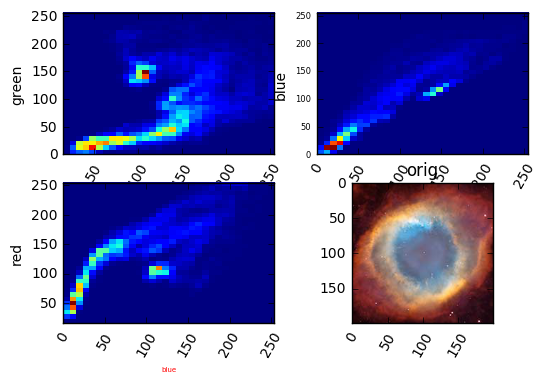In [ ]:


In [ ]:


In [ ]:


In [ ]:


In [ ]:



# Plot with Seaborn

Statistical Plotting with Seaborn

In :
import pandas as pd
import matplotlib.pyplot as plt
import seaborn as sns
%matplotlib inline


### load a dataset online from seaborn¶

In :
tip=sns.load_dataset('tips')

In :
tip.info()

<class 'pandas.core.frame.DataFrame'>
RangeIndex: 244 entries, 0 to 243
Data columns (total 7 columns):
total_bill    244 non-null float64
tip           244 non-null float64
sex           244 non-null category
smoker        244 non-null category
day           244 non-null category
time          244 non-null category
size          244 non-null int64
dtypes: category(4), float64(2), int64(1)
memory usage: 6.8 KB

In :
tip.head(3)

Out:
total_bill tip sex smoker day time size
0 16.99 1.01 Female No Sun Dinner 2
1 10.34 1.66 Male No Sun Dinner 3
2 21.01 3.50 Male No Sun Dinner 3

### visualizing regressions¶

• Plot data and regression model fits across a FacetGrid.
In :
sns.lmplot('total_bill','tip',tip,size=3,aspect=2)

Out:
<seaborn.axisgrid.FacetGrid at 0x7f71804eb950>